# Allocation and Distribution with finaquant® protos

All the source code in C# required for running the examples below including the simple distribution function can be downloaded at the product page of finaquant® protos. See application examples on the product page.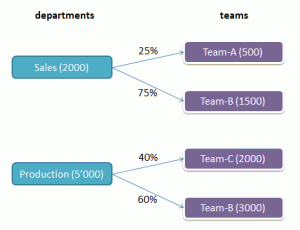Though most commonly used for financial planning and accounting, allocations and distributions can be needed in any calculation where proportionate (pro-rata) distribution of some amounts is required.

A classical distribution example is distributing costs obtained at company level further to departments and teams in proportion to given key amounts or ratios. Another example could be distributing bonus amounts calculated at department level further to teams and individuals.

Increase video quality to 480p if you don’t see the pictures clearly

Distribution is done from a set of entities to other entities. Let’s take distribution of costs from the entity department to entity team as an example. The initial table `costs(department)` is transformed into a bigger table `costs(department, team)` by the distribution with the additional field team.Allocation in turn means distributing some amounts within the same entity group. A typical example is distributing costs from some central cost centers to other decentral cost centers. Note that no additional fields are added into the initial table costs(cost_center) by the allocation; only new field values.

In the examples below, you will see how a distribution can be accomplished in four simple steps using the available table functions in finaquant® protos. These four steps will be than packed into a general distribution function with tables as input and output parameters as shown below: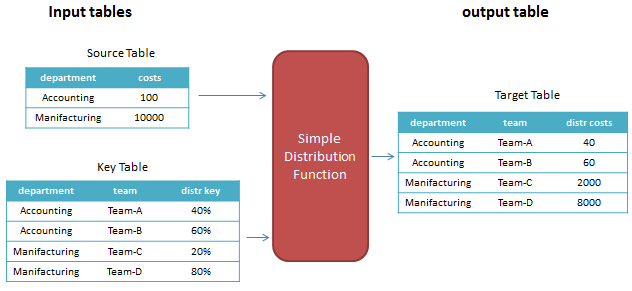The simple distribution function (which is a table function) has two input tables, and an output table, plus some additional detail parameters like the names of new key figures to be added:

`OutputTable = SimpleDistribution(SourceTable, KeyTable, … detail parameters)`

Note that an allocation can be formulated as a special case of distribution, as you will see in the following examples.

## Distribution in four steps: Distribution of costs

In this example, costs are distributed from department to teams and persons. Following cost and key tables are given as inputs:

SourceTable from which the amounts are distributed: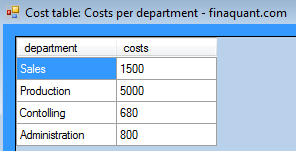KeyTable with distribution keys (or ratios):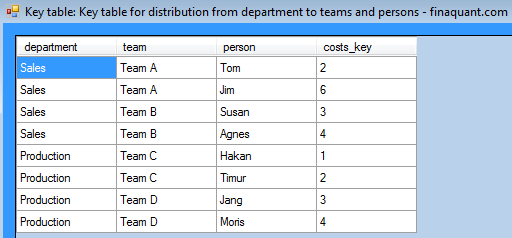The strategy for calculating the distributed costs is the following:

• Ensure that we have every attribute and key figure we need to calculate the distributed costs by combining the tables and adding the necessary key figures into the combined table.
• Calculate the distributed costs by row-by-row processing of combined table.

This is a simple and effective strategy which can be used for many other kinds of table calculations. First, make sure that you have all the required parameters as fields of the table, than calculate the desired fields by row-by-row processing of the table.

### Step 1: Combine cost and key tables

```// combine tables NumVector MatchedRowsTbl1, MatchedRowsTbl2; var CombinedTbl1 = MatrixTable.CombineTables(CostKeyTable, CostTable, null, null, null, out MatchedRowsTbl1, out MatchedRowsTbl2); // filter out unmatched rows CombinedTbl1 = MatrixTable.PartitionRow(CombinedTbl1, MatchedRowsTbl1); // view table MatrixTable.View_MatrixTable(CombinedTbl1, "Step 1: Combined cost and key tables");```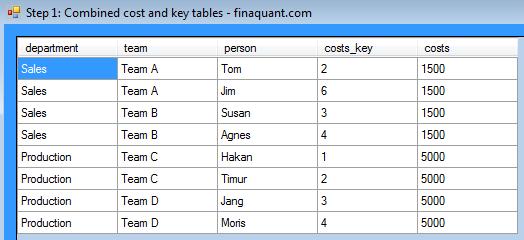We have now two key figures costs and cost_key in the combined table as shown above. The aggregation key figure key_sum (sum of cost_key w.r.t. department, the common attribute of cost and key tables) is still missing. We need key_sum to calculate distributed costs:

### Step 2: Add key figure “key_sum” into the combined table

```bool IfSuccess; string Warnings; // add aggregation key figure into table CombinedTbl1 = MatrixTable.AggregateSelectedKeyFigure_B(CombinedTbl1, TextVector.CreateVectorWithElements("department"), "costs_key", "key_sum", AggregateOption.nSum, out IfSuccess, out Warnings); // view table MatrixTable.View_MatrixTable(CombinedTbl1, "Step 2: Key figure key_sum is added to combined table");```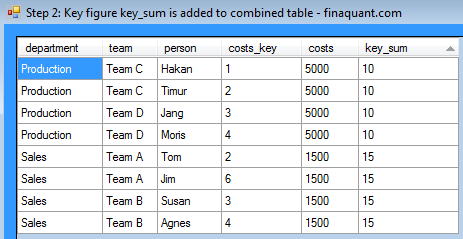### Step 3: Insert new key figure “costs_distributed” into combined table

This key figure must be inserted into the table so that it can be calculated by row-by-row processing of table with the formula stated above.

```// insert key figure "costs_distributed" into combined table CombinedTbl1 = MatrixTable.InsertNewColumn(CombinedTbl1, "costs_distributed", FillAllValue: 0.0); // view table MatrixTable.View_MatrixTable(CombinedTbl1, "Step 3: New key figure costs_distributed is inserted into to combined table");```The initial value of the new key figure costs_distributed is zero for all rows of the combined table. The resultant values for this key figure will be calculated with the next and last step.

### Step 4: Calculate distributed costs

```// row-by-row processing with user-defined function CombinedTbl1 = MatrixTable.TransformRowsDic(CombinedTbl1, DistributeSourceAmountToTarget, "costs_distributed", // target key figure "costs", // source key figure "costs_key", // key "key_sum"); // sum // view table MatrixTable.View_MatrixTable(CombinedTbl1, "Step 4: Combined table after distribution");```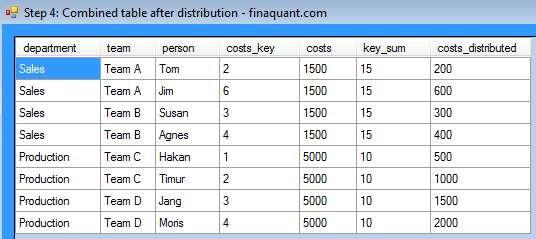User-defined function DistributeSourceAmountToTarget as input to the table transformation function above implements the formula for distributed costs:
```public static void DistributeSourceAmountToTarget( ... // Calculate distributed costs: KeyFigDic[target_keyfig] = KeyFigDic[source_keyfig] * KeyFigDic[key_keyfig] / KeyFigDic[sum_keyfig]; ...```

## Simple Distribution Function

All the four steps for calculating the distributed amounts can be packed into a single general distribution function with the following input and output parameters:
```OutputTable = SimpleDistribution(SourceTable, KeyTable, TargetKeyFig, KeySumKeyFig)```

where:

• TargetKeyFig is the name of the resultant key figure with distributed amounts, like costs_distributed
• KeySumKeyFig is the name of the aggregated key figure as sum of key values w.r.t. common attributes, like key_sum

Following conditions must be satisfied for input parameters of the distribution function:

• Both SourceTable and KeyTable must have exactly one key figure (representing source and key amounts respectively)
• SourceTable and KeyTable must have at least one common attribute (text or numeric)
• Both input tables must share the same MetaData object (i.e. common data universe)
• The key figures TargetKeyFig and KeySumKeyFig must be defined in MetaData
Both input tables must not contain the key figures TargetKeyFig or KeySumKeyFig

The exact signature of the distribution function in C# is as follows:
```public static MatrixTable SimpleDistribution(MatrixTable SourceTable, MatrixTable KeyTable, string TargetKeyFig, string KeySumKeyFig)```Let’s test this function with the same input tables (cost and cost key) introduced in the first example above (distribution in 4 steps):

```MatrixTable CombinedTbl2 = SimpleDistribution(SourceTable: CostTable, KeyTable: CostKeyTable, TargetKeyFig: "costs_distributed", KeySumKeyFig: "key_sum"); // view table MatrixTable.View_MatrixTable(CombinedTbl2, "Result of SimpleDistributuion(): Output table with distributed amounts");```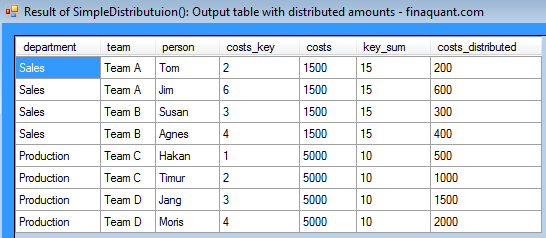So we obtained the same distribution results. That is, the four distribution steps explained in the previous example are successfully packed into a function with generalized input and output parameters. Now you can execute the distribution function on any pair of input tables (source and key) provided that all the parameter conditions listed above are satisfied.

Note that the input tables can have any number of attributes, and they can also have multiple common attributes. In the previous example we had one common attribute: department. In the next example we have multiple common attributes, namely department and country. Source and key amounts are specified with this attribute pair.

## Distributing bonus amounts to teams and years

In this example scenario, a company with headquarters in Paraguay has sales and production departments in countries Bolivia and Peru. The bonus amounts as performance incentives for employees are first calculated per department and country. These bonus amounts are then distributed to teams and years. Bonus amounts are distributed also to years, because the bonus payments will be done at the end of 2013 and 2014.

Source table: Bonus amounts per department and countryKeyTable: Key amounts for the distribution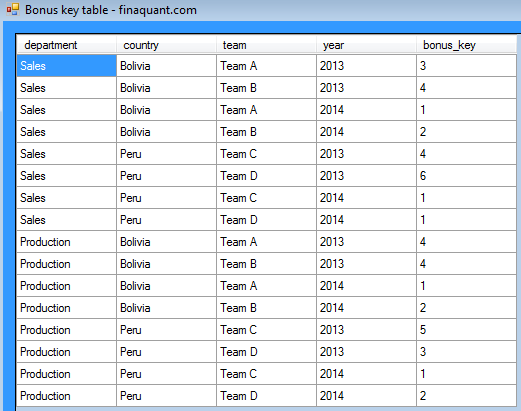The simple distribution function will be tested again with these new input tables:
```// Distribute MatrixTable ResultTbl = SimpleDistribution(SourceTable: BonusTable, KeyTable: BonusKeyTable, TargetKeyFig: "bonus_distributed", KeySumKeyFig: "key_sum"); // view table MatrixTable.View_MatrixTable(ResultTbl, "Result of SimpleDistribution() with bonus and key tables as input: Output table with distributed bonus amounts");```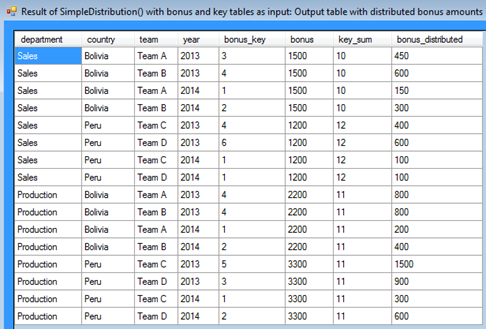Note that the sum of key amounts (key_sum) are obtained by aggregating bonus_key with respect to the attribute pair department and country that are common attributes of input tables.

## Allocating profits to profit centers

This example will illustrate that allocations can be formulated as a special case of distribution.

In this example scenario, the profits accumulated at a main hub are distributed to other cost centers according to the given distribution keys.KeyTable: Key amounts for the allocation of profits to other cost centers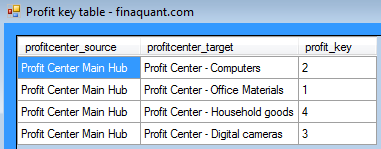Just by separating the attribute profitcenter into two (source and target) we have transformed the allocation into a distribution problem. We can now apply the distribution function on these source and key tables:

```// Distribute ResultTbl = SimpleDistribution(SourceTable: ProfitTable, KeyTable: ProfitKeyTable, TargetKeyFig: "profit_distributed", KeySumKeyFig: "key_sum"); // view table MatrixTable.View_MatrixTable(ResultTbl, "Allocation results with profit and key tables as input: Output table with distributed profits");```## Conclusions

Simple proportionate (pro-rata) distribution can be accomplished by simple table functions in four steps. All these steps can be packed into a general distribution function with two input tables representing the source (initial values to be distributed) and key (distribution ratios) amounts.

Once you have your distribution function, you can forget about the details of the function (four steps etc.) and simply apply this function on any input table pairs specifying the source and key amounts, provided that:

1. You have a simple proportionate (pro-rata) distribution case.
2. All the parameter conditions for the distribution function are satisfied.

There are indeed more complex conditional allocation and distribution cases that cannot be captured by the simple distribution function. Such distribution functions will be offered with the commercial version of finaquant® protos: finaquant® calcs.

Nevertheless, if you have sufficient analytical skills, you can calculate almost any kind of distribution with the table functions of finaquant® protos. The key functions will be table combinations, aggregations and transformations (especially row-by-row processing).

Allocations can be formulated as a distribution problem, as the last example above illustrates (profit allocation).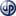Copyright secured by Digiprove © 2012 Tunc Ali Kütükcüoglu
This entry was posted in Calculation engine and tagged , , , . Bookmark the permalink.